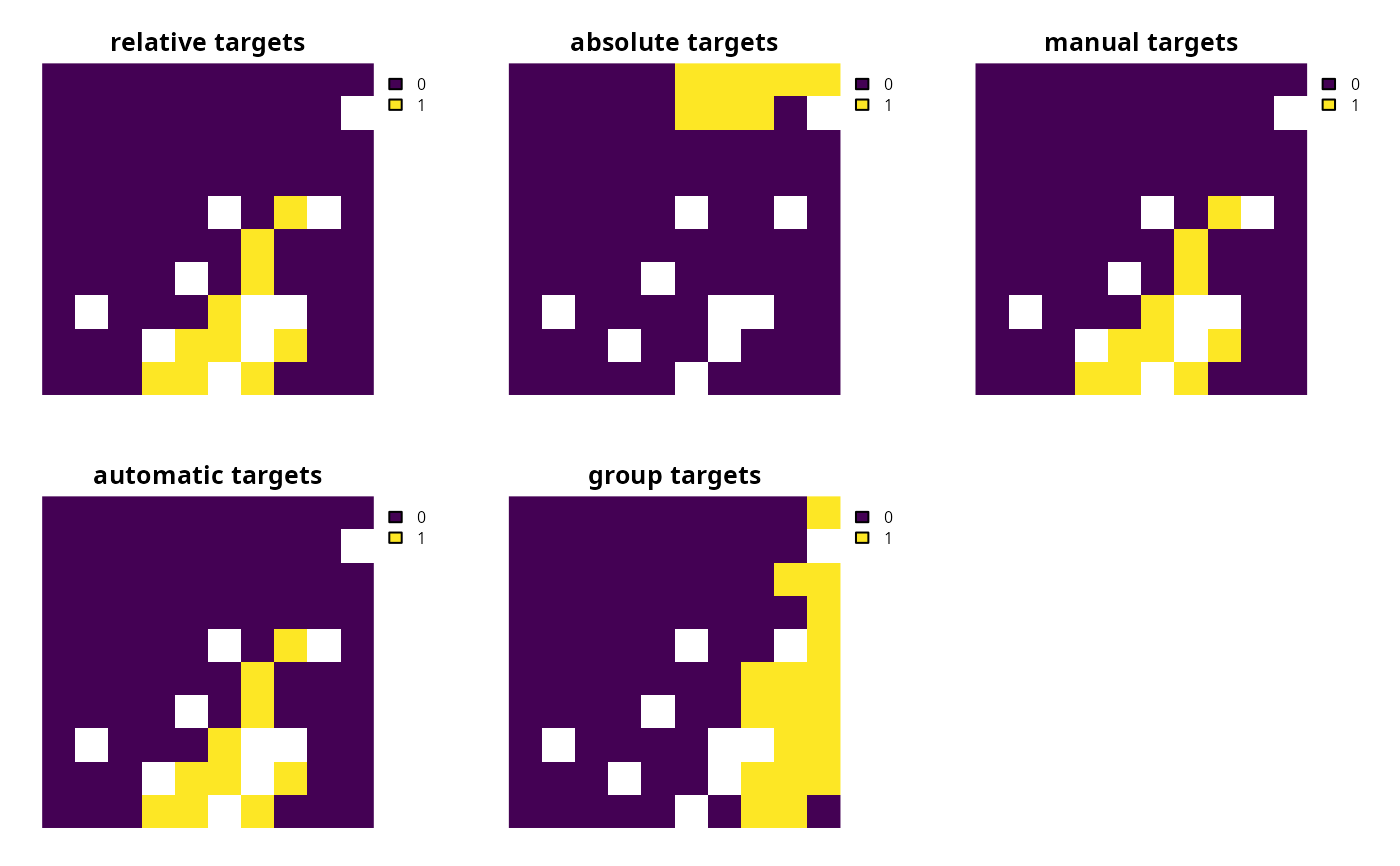Targets are used to specify the minimum amount or proportion of a feature's distribution that should (ideally) be covered (represented) by a solution.

## Details

Please note that most objectives require targets, and attempting to solve a problem that requires targets will throw an error.

The following functions can be used to specify targets for a conservation planning problem():

add_relative_targets()

Set targets as a proportion (between 0 and 1) of the total amount of each feature in the the study area.

add_absolute_targets()

Set targets that denote the minimum amount of each feature required in the prioritization.

add_loglinear_targets()

Set targets as a proportion (between 0 and 1) that are calculated using log-linear interpolation.

add_manual_targets()

Set targets manually.

Other overviews: constraints, decisions, importance, objectives, penalties, portfolios, solvers, summaries

## Examples

# \dontrun{
sim_pu_raster <- get_sim_pu_raster()
sim_features <- get_sim_features()

# create base problem
p <-
problem(sim_pu_raster, sim_features) %>%

# create problem with added relative targets

# create problem with added absolute targets

# create problem with added loglinear targets
p3 <- p %>% add_loglinear_targets(10, 0.9, 100, 0.2)

# create problem with manual targets that equate to 10% relative targets
targs <- data.frame(
feature = names(sim_features),
target = 0.1,
type = "relative"
)